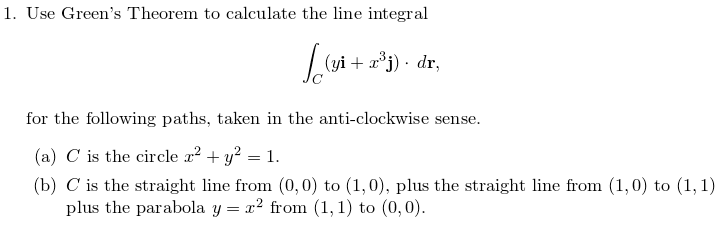# Green's Theorem Homework - Solve for ∫c (3x2 -1).dr

• gl0ck

## Homework Statement

Hello,

I know this might be trivial but,
can you please tell me what I am missing?
Here is my problem :## Homework Equations

Given F = (yi+x3j).dr
Q = x3
P = y
=> ∂Q/∂x = 3x2 and ∂P/dy = 1
c (3x2 -1).dr = ∫2Pi010 (3r2cosθ2-1) rdrdθ
2Pi010 (3r3cosθ2-r) drdθ
Is it true so far ?

Thanks

Last edited:

Hi,

What you've done is right. Go ahead with the problem.

Hi,

What you've done is right. Go ahead with the problem.

2Pi010 (3r3cosθ2-r) drdθ
->∫2Pi0(3/4cos2θ-1/2) dθ
->3/4∫2Pi0(cos2θ-1/2) dθ
->3/8[θ+sin(θ)cos(θ)-θ/2]2Pi0
->-Pi/4

Is this now correct?
If it is correct what about part b)
it looks to me like 1/4 circle when it is plotted but here I have y = x^2 and no radius to start the same thing again and I am not sure about the 1st integral neither, becuase I think it cannot be from 0 to Pi/2 because it doesn't start from x= 0 but from y = 0

Last edited:
2Pi010 (3r3cosθ2-r) drdθ
->∫2Pi0(3/4cos2θ-1/2) dθ
->3/4∫2Pi0(cos2θ-1/2) dθ
->3/8[θ+sin(θ)cos(θ)-θ/2]2Pi0
->-Pi/4

Is this now correct?

If it is correct what about part b)
it looks to me like 1/4 circle when it is plotted but here I have y = x^2 and no radius to start the same thing again and I am not sure about the 1st integral neither, becuase I think it cannot be from 0 to Pi/2 because it doesn't start from x= 0 but from y = 0

Stick to cartesian coordinates on this one. What are the limits on y (in terms of x) and x?

Stick to cartesian coordinates on this one. What are the limits on y (in terms of x) and x?

Can we take that r = 1 because the line from (0,1) to (1,1) is the radius if we take it as 1/4 of a circle and this is the bottom right part?
A bit confused by the limits though. I think x is from 0 to $\sqrt{}y$
and y is from 0 to 1?

No, there is no "quarter circle". To integrate over the region, take x going from 0 to 1 and, for each x, y going from $x^2$ to x.

(You could do in the other order, taking y from 0 to 1 and, for each y, x from y to $\sqrt{y}$.)

No, there is no "quarter circle". To integrate over the region, take x going from 0 to 1 and, for each x, y going from $x^2$ to x.

(You could do in the other order, taking y from 0 to 1 and, for each y, x from y to $\sqrt{y}$.)

Isn't it supposed to be y from 0 to x2 and and x from 0 to 1 ?

Alternatively, x from √y to 1 and y from 0 to 1.

Isn't it supposed to be y from 0 to x2 and and x from 0 to 1 ?

Alternatively, x from √y to 1 and y from 0 to 1.

With these boundaries I found it to be 4/15 which is the answer indeed
Thanks very much !
Can I ask you one more thing, when if ever we can refer to a circle to solve these kind of questions, or I should look for x^2 + y^2 = r , in order to refer to a circle?

when if ever we can refer to a circle to solve these kind of questions, or I should look for x^2 + y^2 = r , in order to refer to a circle?

Yes that's right. Only if your boundary is a circle can you refer to a circle. You must use the equation of the boundary given. Here, for example, the boundaries were y=x2, y=0 and x=1.